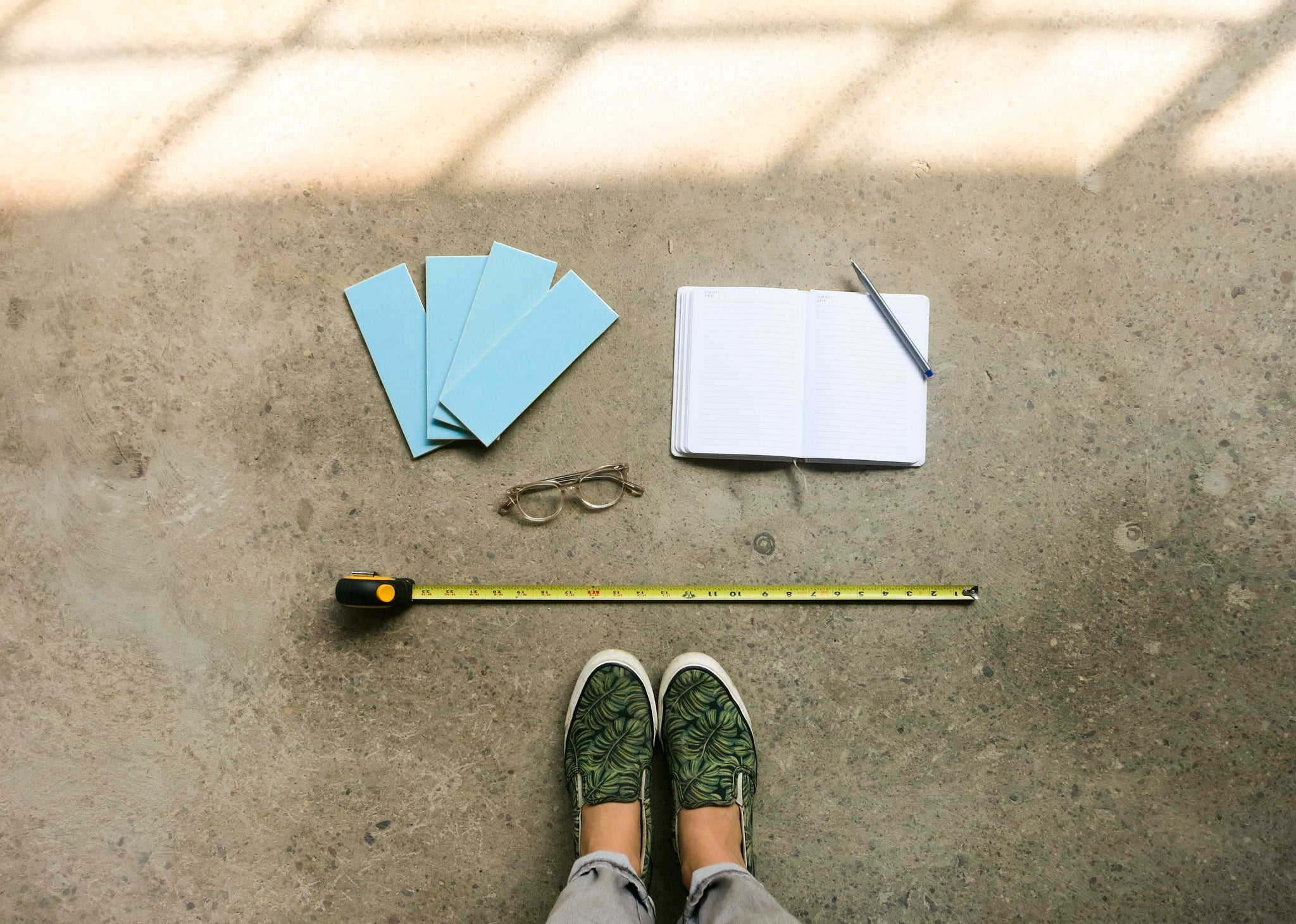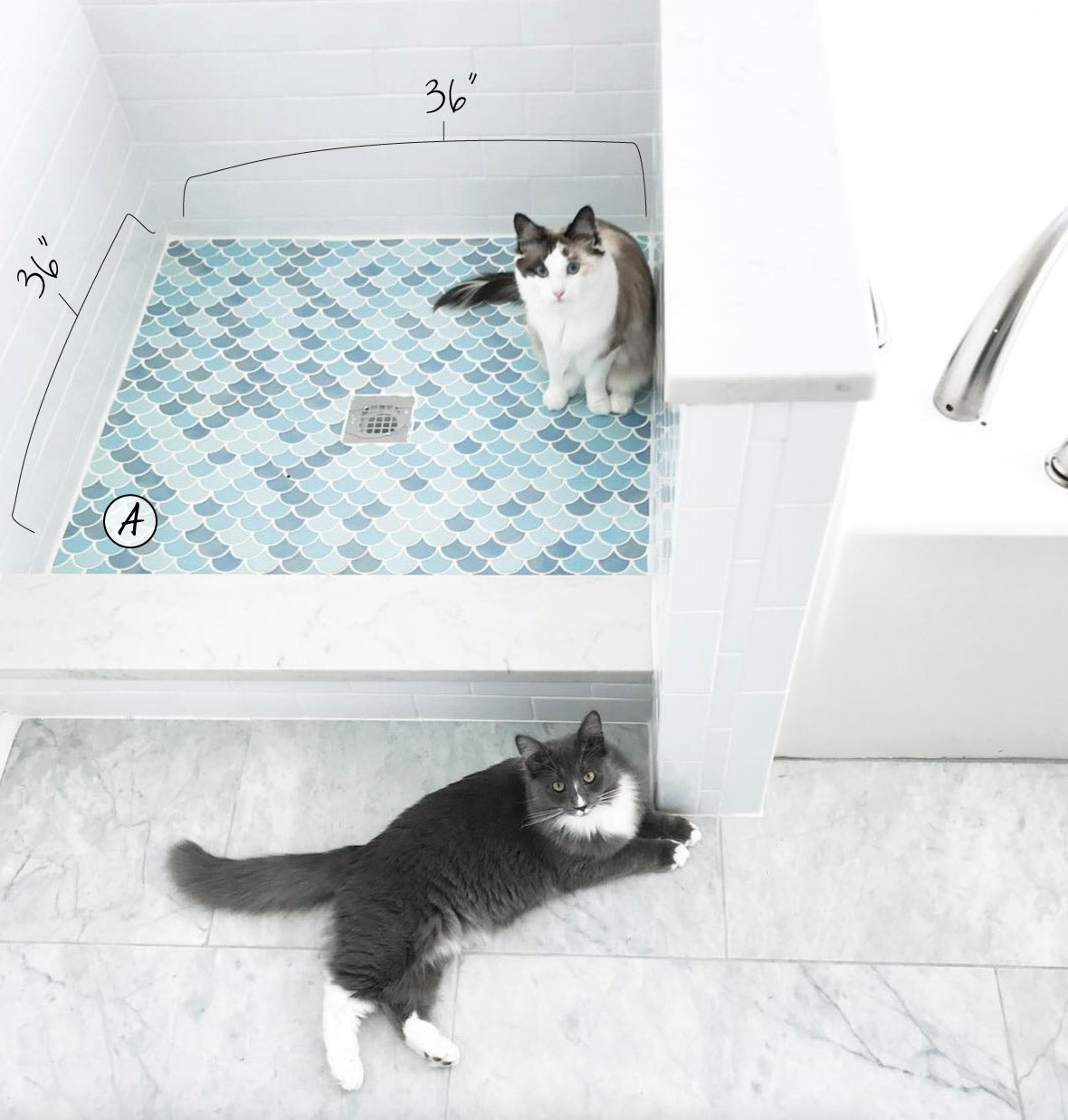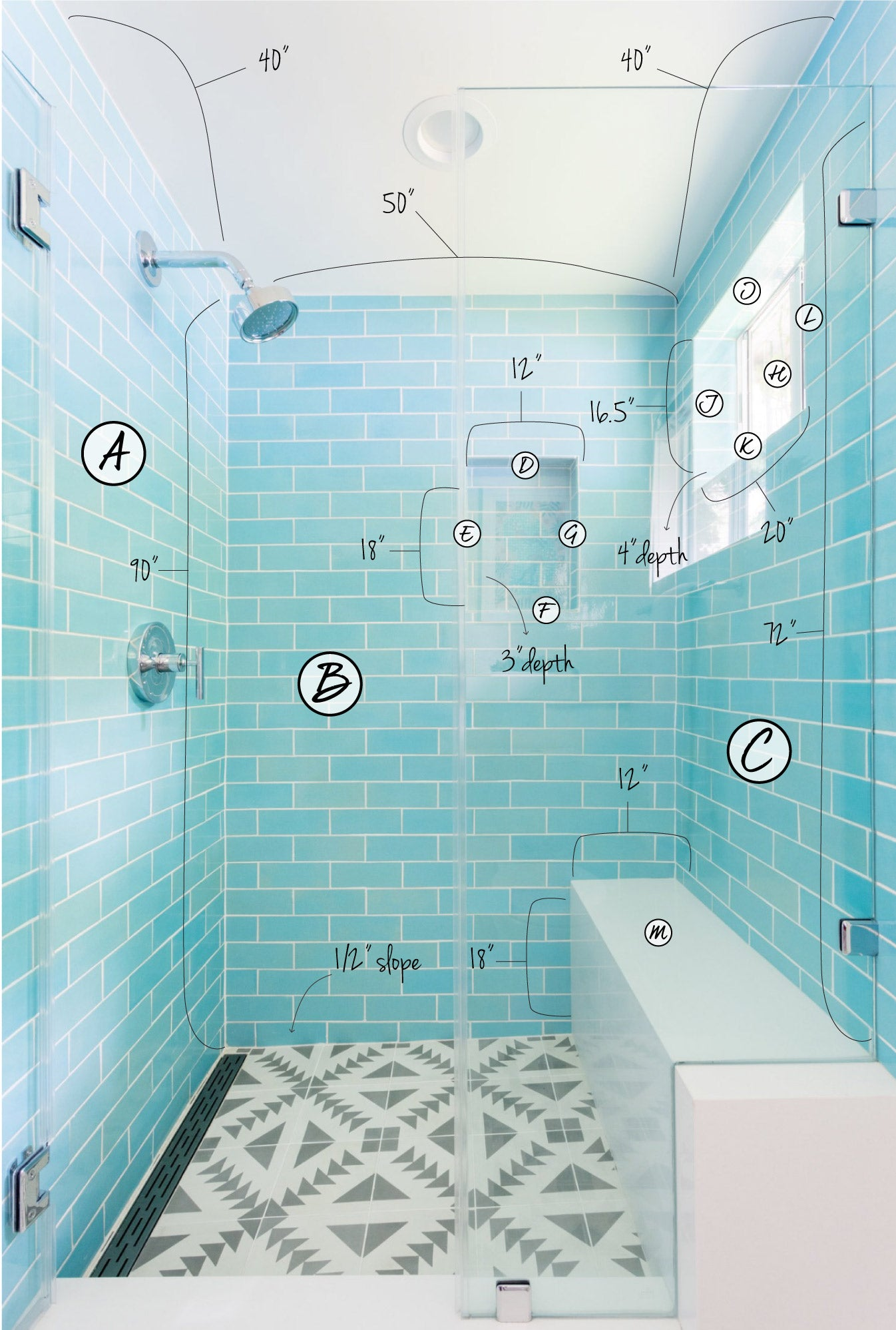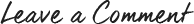# How Much Tile Do I Need? Calculating Tile Needed for Your Bathroom

In every bathroom remodel project, you will eventually have to ask the question, "How much tile do I need?" Maybe you are trying to figure out your budget for bathroom tile. Or are you ready to order from our online shop but don’t know how to calculate tile needed for your bathroom? This blog is here to help simplify the process of measuring and calculating your tile area!

## What do I need to measure my tile area?

• Paper
• Writing utensil
• Calculator
• Tape measure
• Photo of the space (optional)
• Samples of your tile (optional) Order samples here!## How do I calculate the tile needed for my bathroom?

This task can be easy to overcomplicate, but it's really quite simple! We've even made a free downloadable worksheet for you to work from. In this blog we're outlining three examples of how to calculate the tile needed for a floor, bathroom backsplash, and a shower with a niche.

## Here are seven steps to measuring your tile area:

1. Draw or print a photo of the space.
2. Measure every side in inches and mark the drawing or photo.
3. If needed, break the spaces into sections, labeling each with a capital letter  from A-Z to keep them from getting confused.
4. Multiply the height and width of each section.
5. Add together all the sections and divide that number by 144 for the square footage.
6. Subtract windows or benches from the total as appropriate.
7. Add 10% overage, or 20% for our Moroccan Fish Scales (multiply by 1.1 or 1.2).

## Example #1 - How much tile do I need for my floor?

Floors are the easiest, we only need to create a section A. Here's our math for the example below:

• Section A
• 36"x36"=1,296
• 1,296/144 =9 square feet
• (add overage) 9x1.2=10.8 square feet

Additional factors to keep in mind are your drains, vanities, toilets or other items that take up space on the floor. Most items will be removed during demolition, we always recommend tiling underneath items for future updates to the bathroom. If you have an item that cannot be removed, simply turn your floor into two areas rather than one.Small Moroccan Fish Scales: 1064 Baby Blue, 45W My Blue Heaven, 43 Robin's Egg.

## Example #2 - How much tile do I need for my bathroom backsplash?

Bathroom backsplashes often have a mirror breaking the rectangular area into multiple sections. There may also be cabinetry that creates additional sections. Here's how we'd draw out and measure the square footage in this situation.

• Section A
• 15.25"x33.125"=505.16
• Section B
• 4.75"x67"=318.25
• Section C
• 15.25"x33.125"=505.16
TOTAL AREA:
• Section A + Section B + Section C = 1,328.57
• 1,328.57/144 = 9.2 square feet
• (add overage) 9.2x1.1=10.12 square feet## Example #3 - How much tile do I need for my shower?

Showers can easily be three rectangular sections, but if you have a niche, that's a few extra sections to keep track of. While it's more to calculate, our labeling system will help your calculations stay organized and simple. This is sort of the "expert level" of calculating your tile space. If it gets too confusing, simply send the measurements to our team and we'll help find the right amount of tile to purchase!

The only trick is to subtract sections where tile will not be placed. In the example below, for Section B we need to subtract the shower bench or Section M. For Section C we need to subtract the window or Section H.

 Section A 90"x40"=3,600" Section H 16.5"x20"=330" Section B 90"x50"=4,500" Section I 20"x4"=80" Section C 72"x40"=2,880" Section J 16.5"x4"=66" Section D 12"x3"=36" Section K 20"x4" =80" Section E 18"x3"=54" Section L 16.5"x4" =66" Section F 12"x3"=36" Section M 18"x12"=216" Section G 18"x3=54"

TOTAL AREA:

• Add all sections excluding the bench and window (Sections M & H) = 11,452
• Now subtract the areas where there is no tile (Sections M & H):  11,452 - 330 -216 = 10,906
• 10,906"/144 = 75.7 square feet
• 75.7x1.1=83.3 square feet3″x8″ Subway Tile – 12W Blue Bell. Photo & Interior Design by Marissa Cramer.

## Need to Measure a Kitchen Backsplash? Read this blog here.##Order tile samples to see, touch, and fall in love with our handmade tiles in person. We would also love to hear what inspires you! Tell us more about your future project and get the ball rollingInspiration from our latest handmade tile projects and ideas for designers, architects and homeowners-alike.

### Behind the Scenes## Get a Free Quote

Tell us: shape, color, quantity, design notes and anything else you want us to know about the tile project you're working on and we’ll get back to you - supporting you with the information you need to continue your tile project planning.